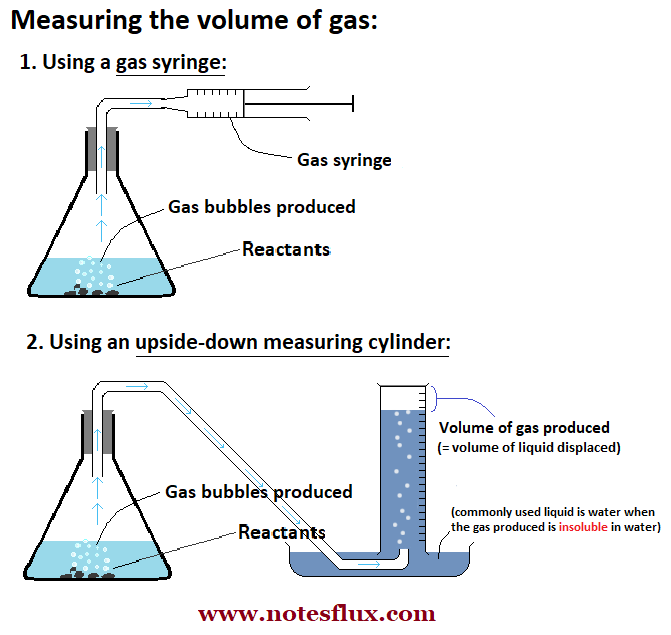# Rates of Reaction:

The term "rate of reaction" means how quickly (or slowly) a reaction happens. It also tells you at what rate the reactants are used up, or the products are formed in a chemical reaction.

For calculating the rate of a chemical reaction, you will need the following:

1. The mass (or the volume) of the reactants (or the products) at the beginning and the end of the reaction.

2. The total amount of time for the reaction.

Once you have both the values, just divide the change in the mass (or the volume) by the total time taken for the reaction. This will be the rate of the reaction.

The rate of reactions is usually measured in:

• For change in mass: Grams per second (g s-1), or

• For change in volume: Cubic decimetre per second (dm3 s-1)

Important: Rate of reaction can also be measured in moles per second (Mol s-1). For this, you will need to convert the given mass or volume to number of Moles.

In order to calculate the number of moles in a given mass of reactants or products, you will be need to be able to calculate their relative formula mass (Mr). The following image shows how to calculate relative formula mass, with an example:

Important: You need to be able to accurately measure the amount of reactants (or the products) to calculate the rate of reaction. You can either measure the mass or the volume of the reactants (or the products). The image below shows two ways of measuring the volume of the gases produced in a chemical reaction.Fig 2. Measuring the volume of a gas produced in a chemical reaction

## The collision theory of chemical reactions:

According to the collision theory, the reactant particles must collide with each other, with enough energy, for a reaction to take place. A collision of reactant particles that results in a reaction is called a successful reaction.

### 1. Effect of temperature:

Increasing the temperature increases energy of the particles and the frequency of collisions; this results in an increase in the rate of reaction.

### 2. Effect of concentration:

When the concentration of a reacting solution or the pressure of a reacting gas is increased, the particles are closer, and, as a result, the collisions between the reactant particles increase.

Higher concentration increases the rate of reaction.

### 3. Effect of surface area:

The larger the surface area, the more particles are exposed and the frequency of collisions between particles increases and the rate of reaction increases.

### 4. Effect of a catalyst:

A catalyst is a substance that speeds up the rate of a reaction. Catalyst is not chemically changed or used up during the reaction.

Biological catalysts used in our bodies are called enzymes.

Catalysts increase the rate of reaction by providing a different pathway for the reaction that requires less energy.

The minimum energy the particles need in order to react is called the activation energy.